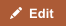Capital expenses are either amortized or depreciated depending upon the type of asset acquired through the expense. Tangible assets are depreciated over the useful life of the asset whereas intangible assets are amortized.

Comparison chart

Amortization versus Depreciation comparison chartAmortizationDepreciation
What is it? A way to "recover costs" i.e. account for capital expenditures. A way to "recover costs" i.e. account for capital expenditures.
Use for Intangible assets e.g. patents Tangible assets e.g. machinery

Cost Recovery

When a business spends money to acquire an asset, this asset could have a useful life beyond the tax year. Such expenses are called capital expenditures and these costs are "recovered" or "written off" over the useful life of the asset. If the asset is tangible, this is called depreciation. If the asset is intangible; for example, a patent or goodwill; it's called amortization.

To depreciate means to lose value and to amortize means to write off costs (or pay debt) over a period of time. Both are used so as to reflect the asset's consumption, expiration, obsolescence or other decline in value as a result of use or the passage of time. This applies more obviously to tangible assets that are prone to wear and tear. Intangible assets, therefore, need an analogous technique to spread out the cost over a period of time. In the United States, intangible property which is subject to amortization must be described in 26 U.S.C. §§ 197(c)(1) and 197(d) and must be property be held either for use in a trade, business, or for the production of income. Under §197 most acquired intangible assets are to be amortized ratably over a 15-year period. If an intangible is not eligible for amortization under § 197, the taxpayer can depreciate the asset if there is a showing of the assets useful life. 

Depreciation or Amortization Schedule

As an example, suppose in 2010 a business buys \$100,000 worth of machinery that is expected to have a useful life of 4 years, after which the machine will become totally worthless (a residual value of zero). In its income statement for 2010, the business is not allowed to count the entire \$100,000 amount as an expense. Instead, only the extent to which the asset loses its value (depreciates) is counted as an expense.

Straight Line Depreciation

The simplest way to depreciate an asset is to reduce its value equally over its life. So in our example, this means the business will be able to deduct \$25,000 each in the income statement for 2010, 2011, 2012 and 2013.

Accelerated Depreciation

Accelerated depreciation methods provide for a higher depreciation charge in the first year of an asset's life and gradually decreasing charges in subsequent years. This may be a more realistic reflection of an asset's actual expected benefit from the use of the asset: many assets are most useful when they are new. A popular accelerated method is the declining-balance method. Under this method the depreciation is calculated as:

Annual Depreciation = Depreciation Rate * Book Value at Beginning of Year

In our example, let's say the business decides to use a depreciation rate of 40%.

Book value at
beginning of year
Depreciation
rate
Depreciation
expense
Accumulated
depreciation
Book value at
end of year
\$100,000 (original cost) 40% \$40,000 \$40,000 \$60,000
\$60,000 40% \$24,000 \$64,000 \$36,000
\$36,000 40% \$14,400 \$78,400 \$21,600
\$21,600 40% \$8,640 \$87,040 \$12,960
\$12,960 Not applicable
(last useful year)
\$12,960 \$100,000 Zero